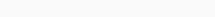# Math Puzzles Puzzle 75 Answer AndroidMath puzzle game level 75 solution with detailed hints and explanation, Scroll below to find out.

Math puzzles increase your logical thinking. The objective of the game is to find patterns hidden in the puzzle in order to arrive at the solution. Challenge yourself with different levels of math puzzles and stretch the limits of your intelligence.

### Math Puzzles Puzzle 75:

if 4,4=6

7,11=6

1,4=5

0,17=4

then 10,44=?Solution/Hint: count total number of straight lines in digits

like in this question 4 have 3 lines

1 have 2 lines

7 have 2 lines 0 have 0 lines

2+0+3+3=8Yay solved all levels, Thank you for visiting this site.

If there is any Doubt or you are unable to understand the solution, then please let me know in comments, I will surely help you.

### One thought on “Math Puzzles Game Level 75 Answer with Solution”

•May 14, 2021 at 15:21